# NCERT Solutions Class 10 Maths Chapter 3 Pair Of Linear Equations In Two Variables

Here you can get free NCERT Solutions for Class 10 Maths, of Chapter 3 Pair Of Linear Equations In Two Variables. All NCERT Book Solutions are given here exercise wise for Pair Of Linear Equations In Two Variables. NCERT Solutions are helpful in the preparation of several school level, graduate and undergraduate level competitive exams. Practicing questions from NCERT Maths Book, its Solutions for Class 10 Chapter 3 Pair Of Linear Equations In Two Variables is proven to enhance your subject skills.

 Class: 10th Class Chapter: Chapter 3 Name: Pair Of Linear Equations In Two Variables Subject: Maths

## NCERT Solutions Class 10 Maths Chapter 3 – Pair Of Linear Equations In Two Variables

NCERT Class 10 Solutions Chapter 3 – Pair Of Linear Equations In Two Variables are given below.

NCERT Solutions for Class 10 Maths Chapter 3 Pair of Linear Equations in Two Variables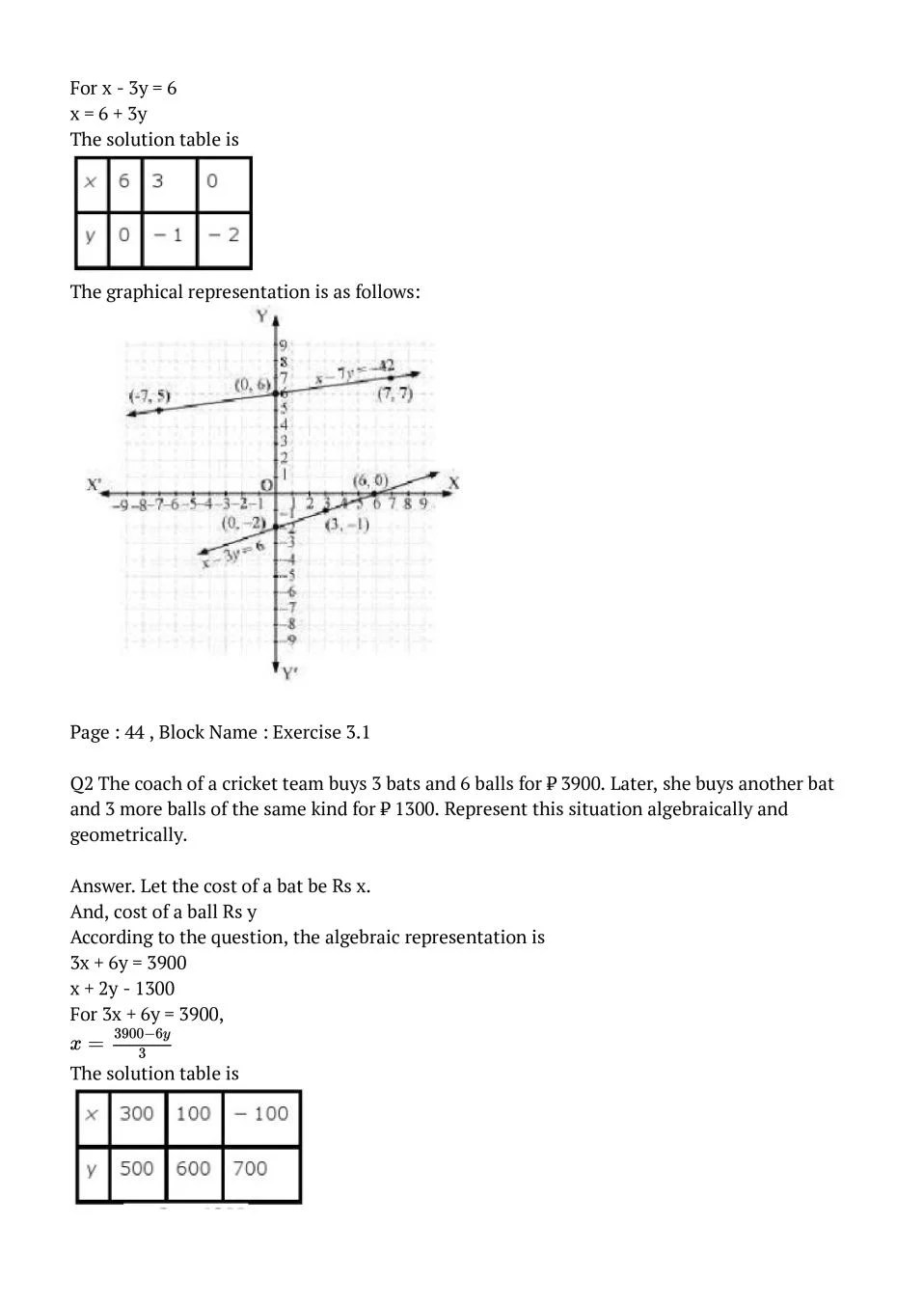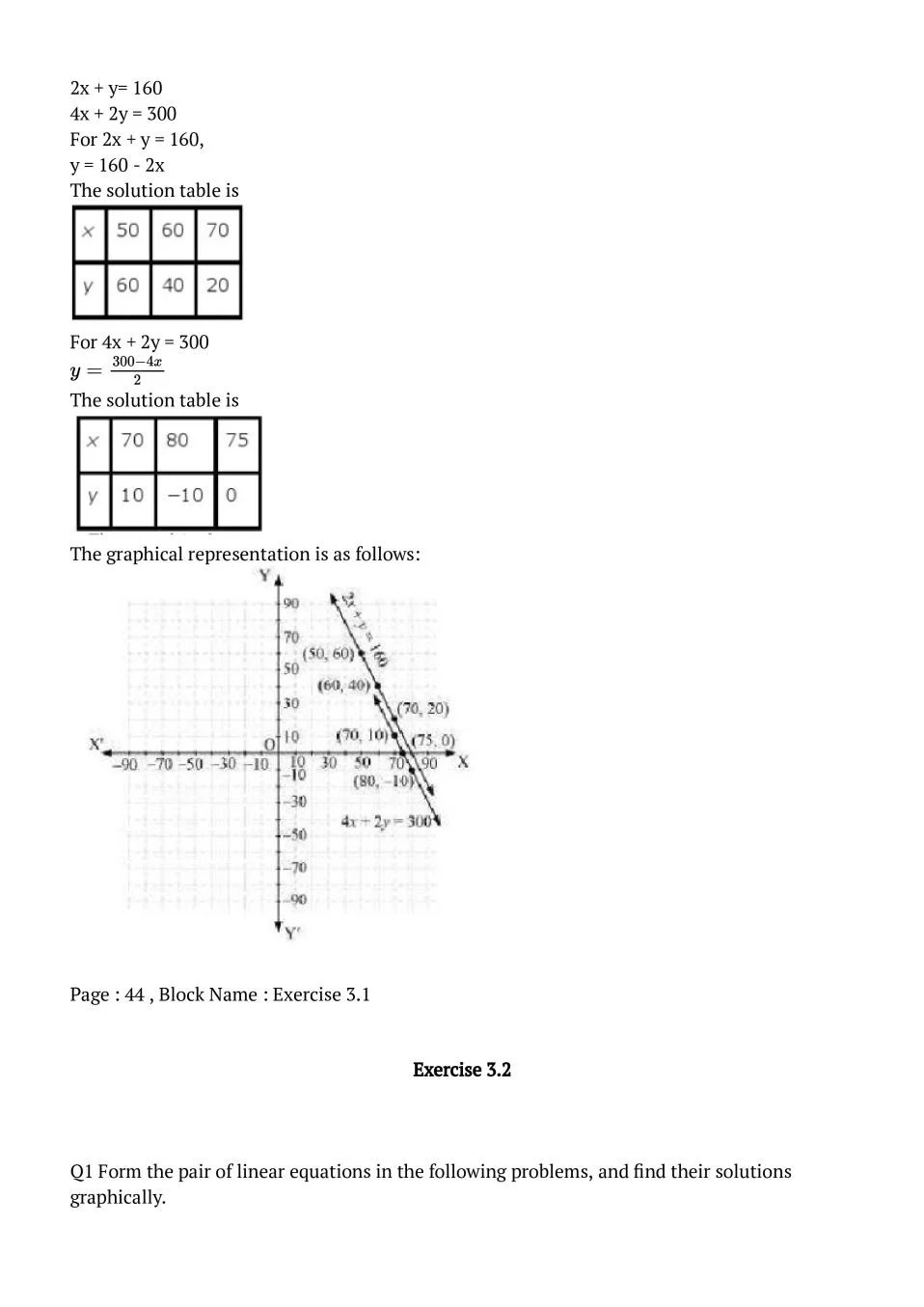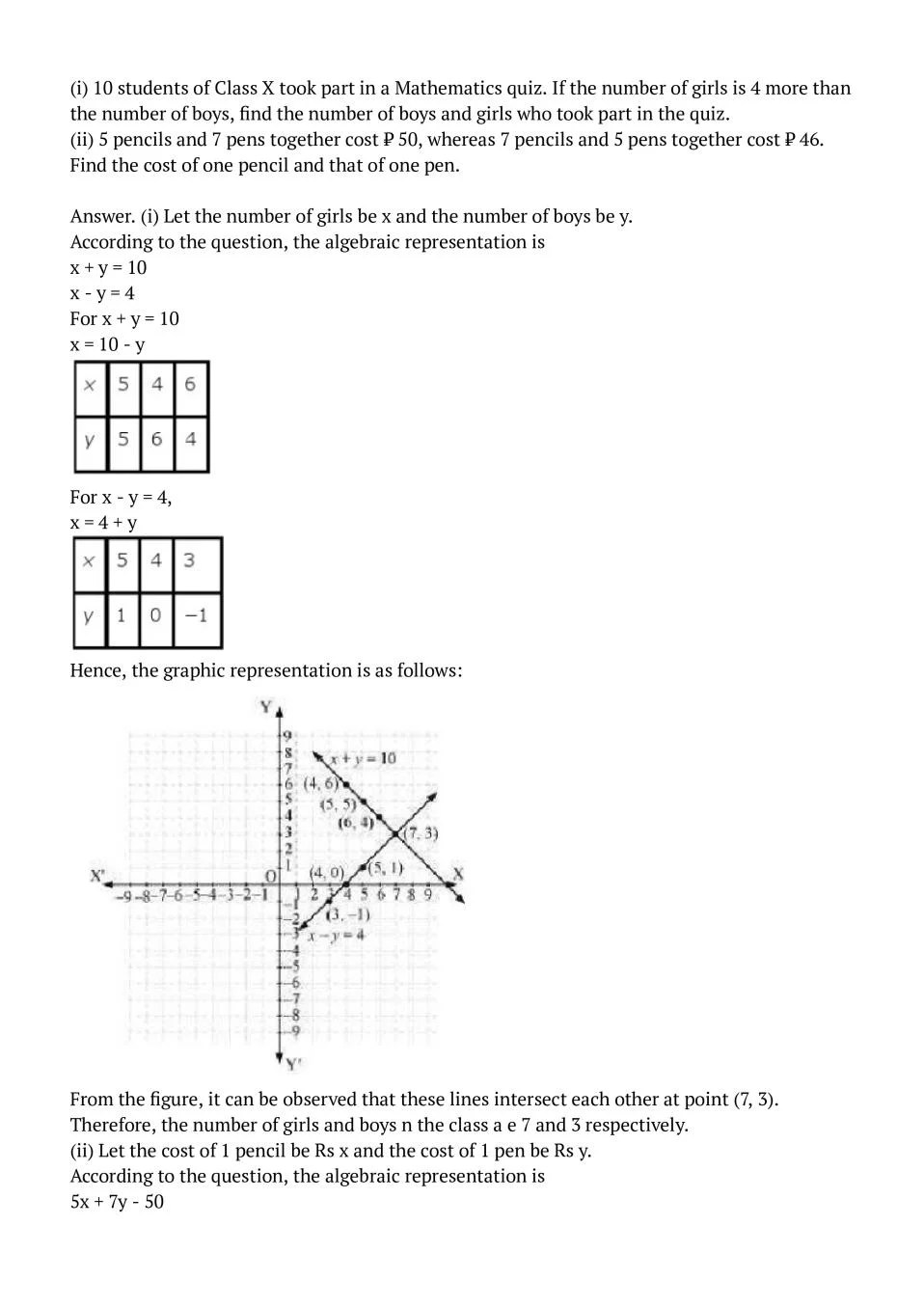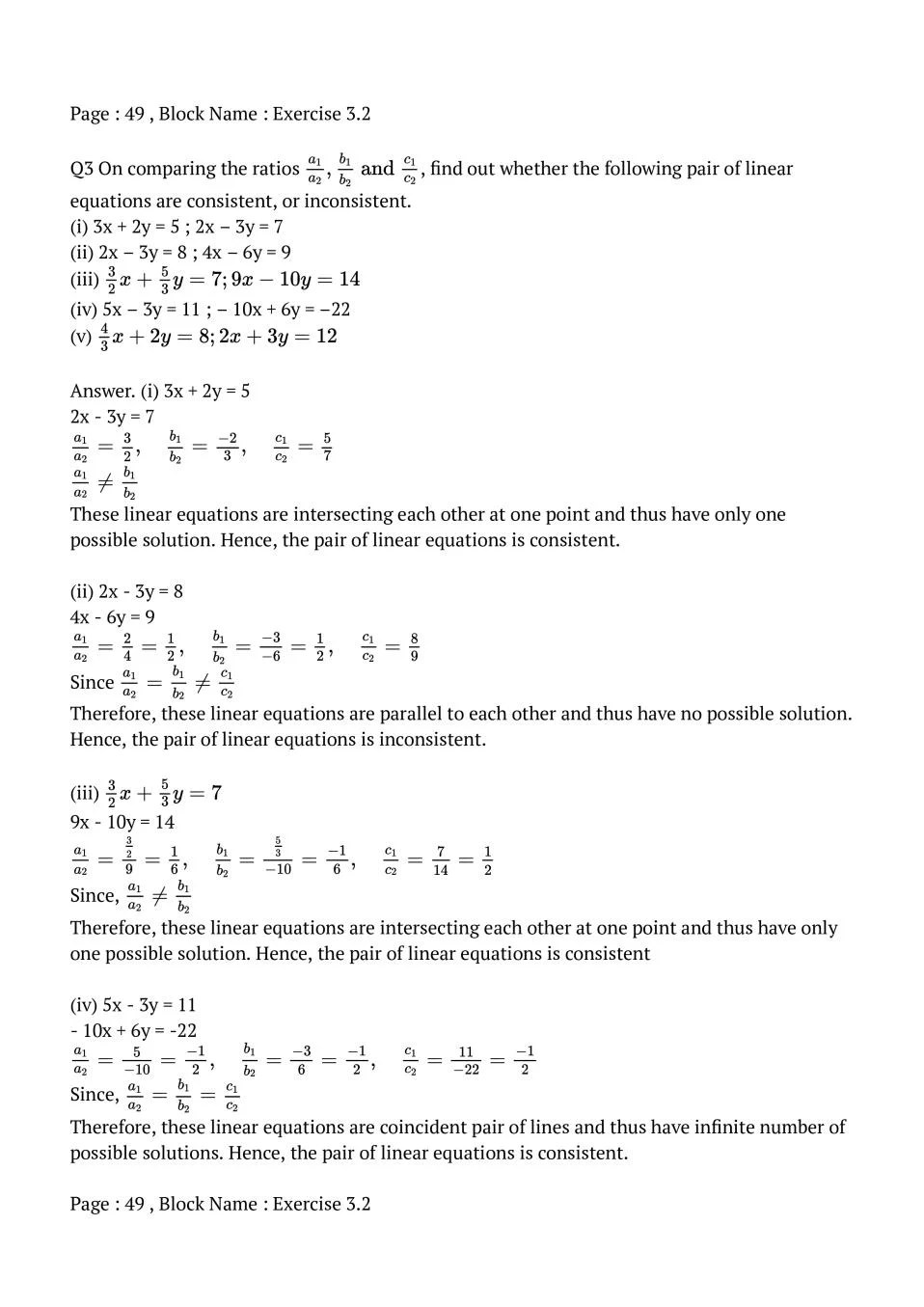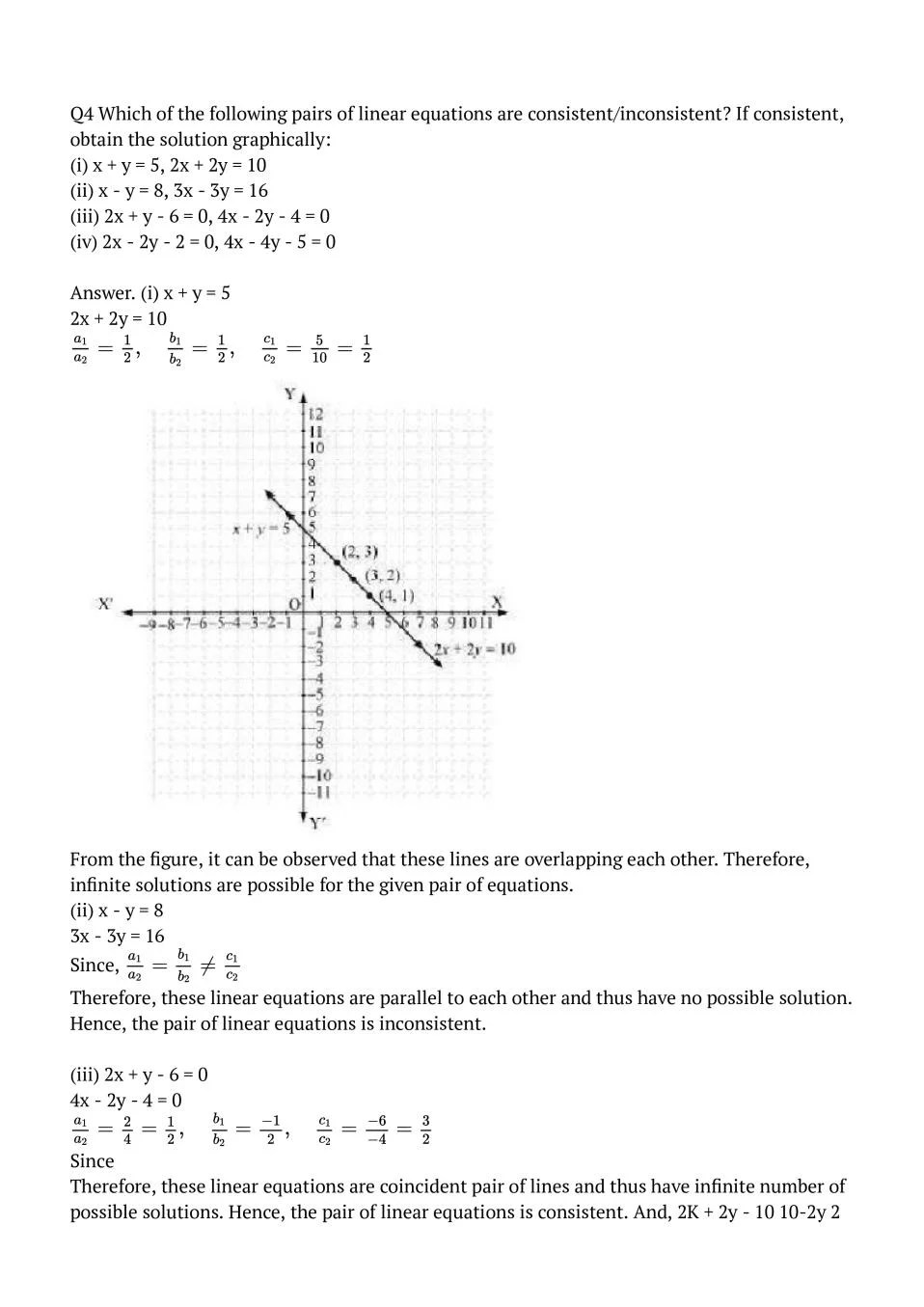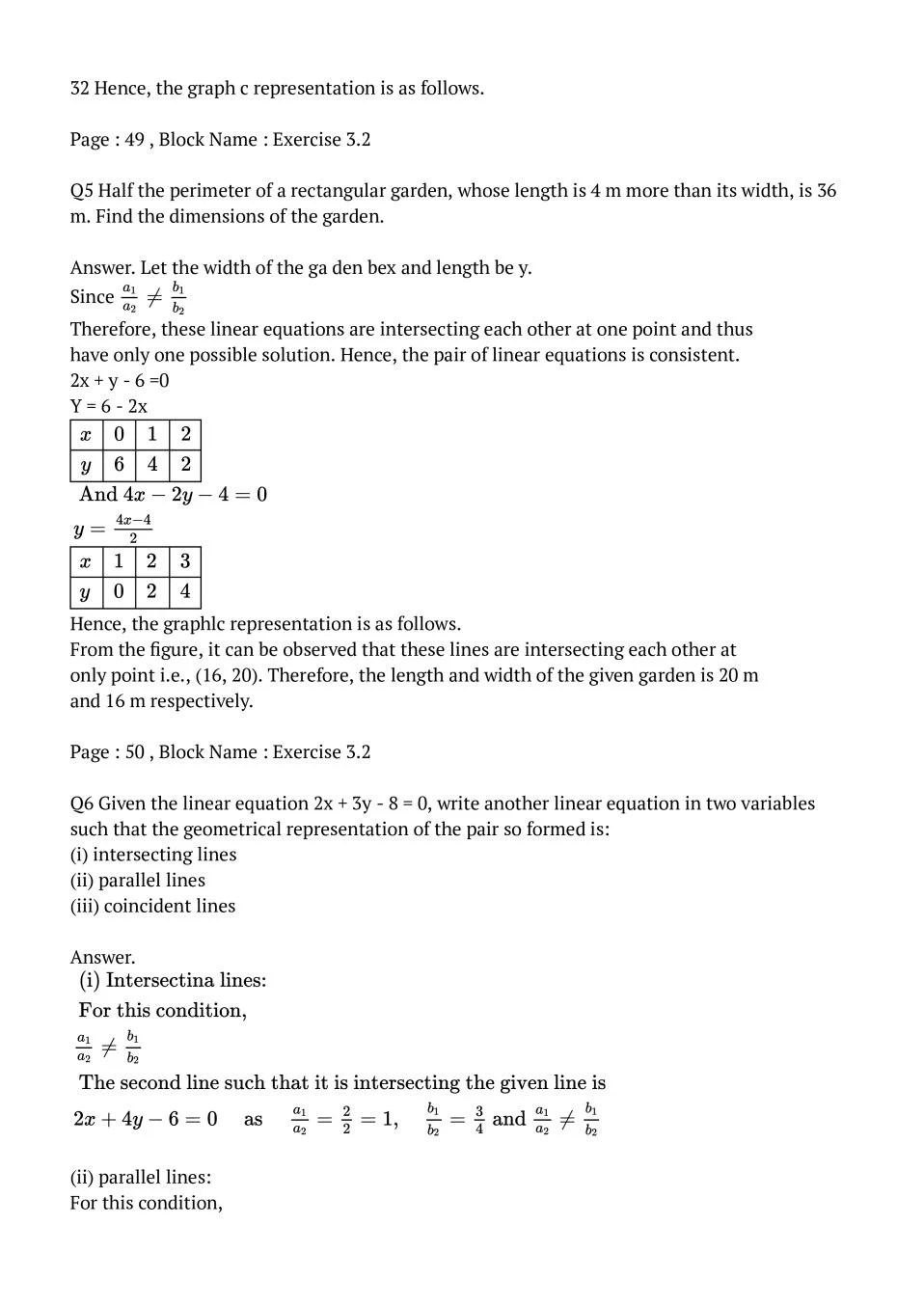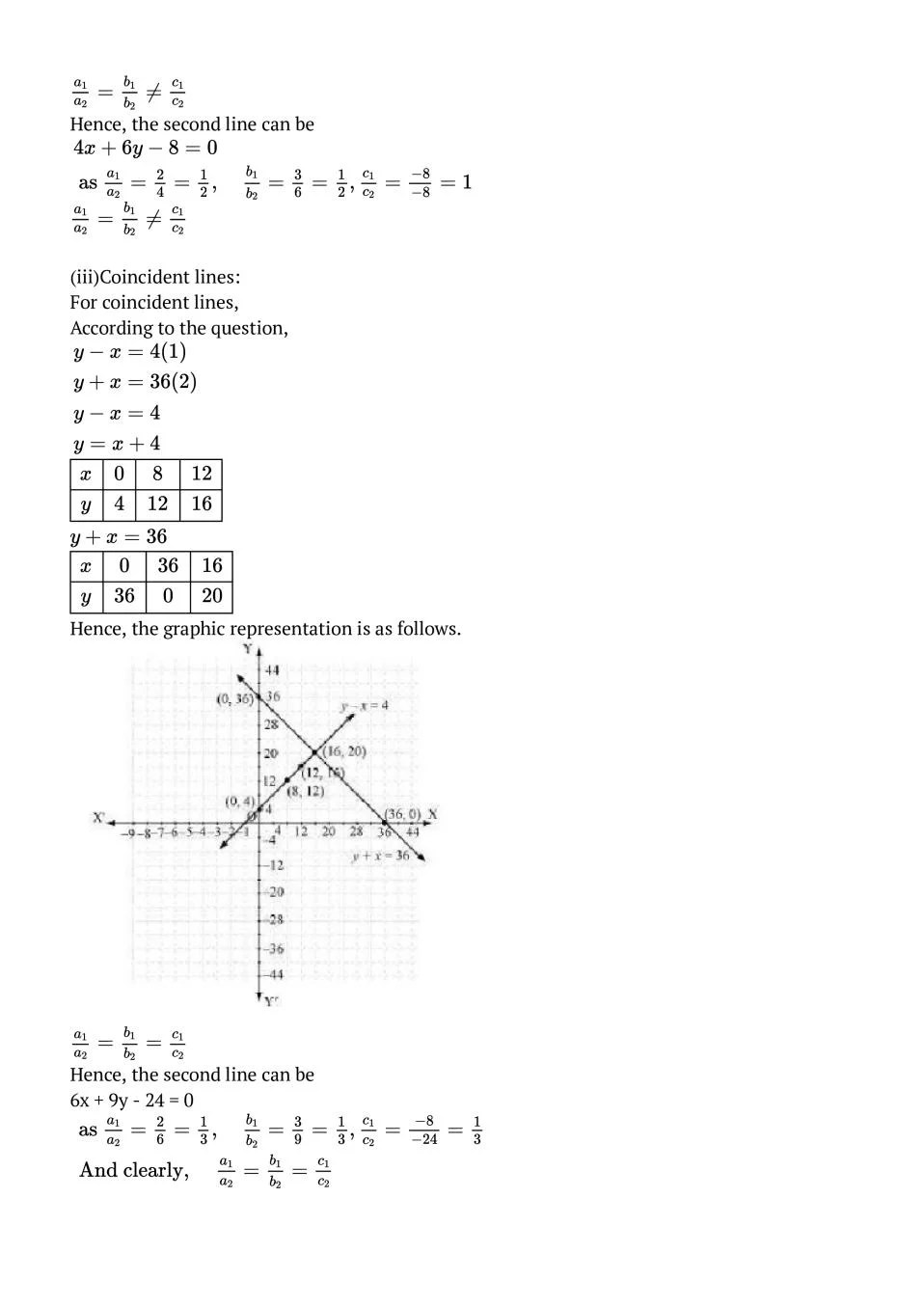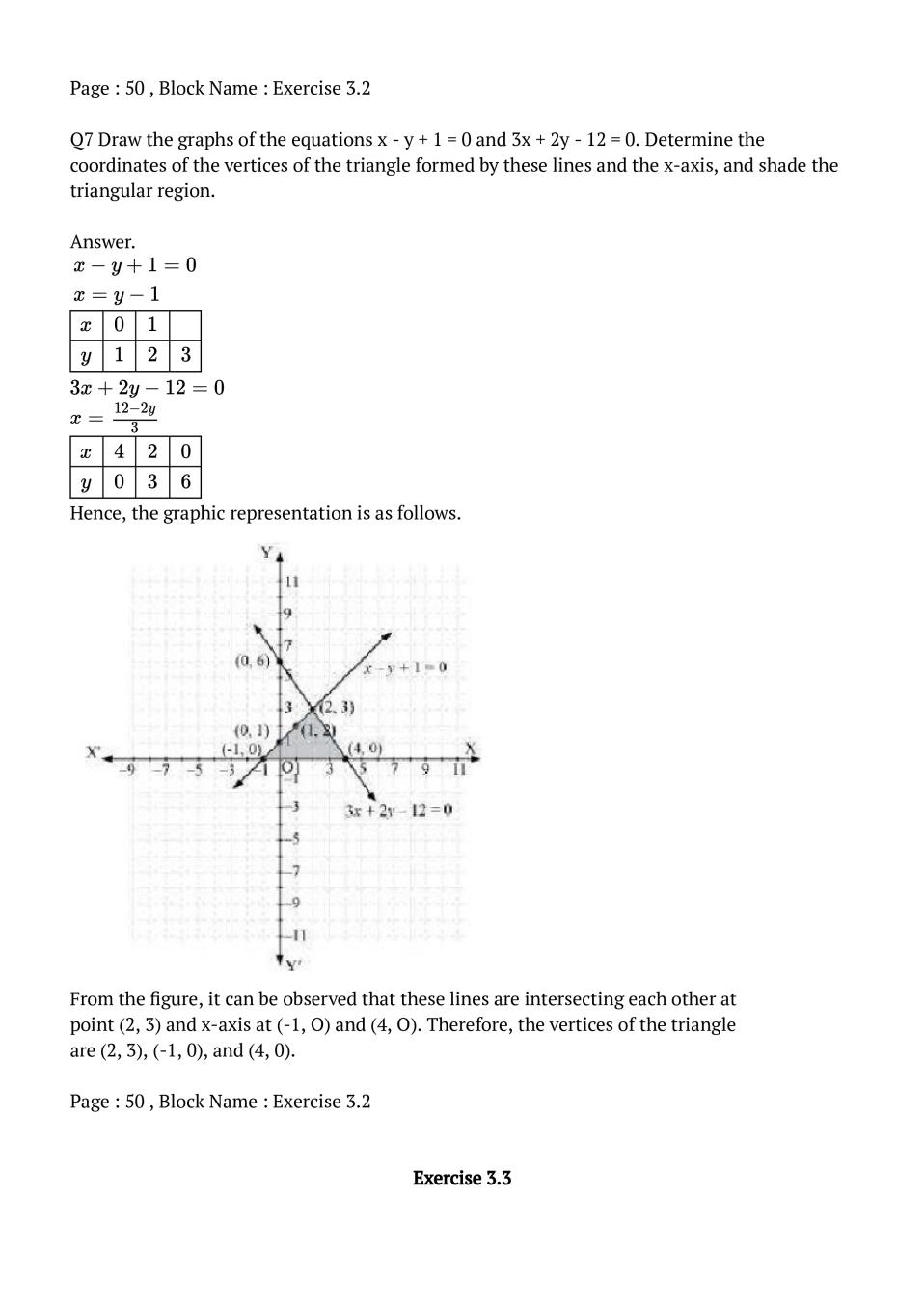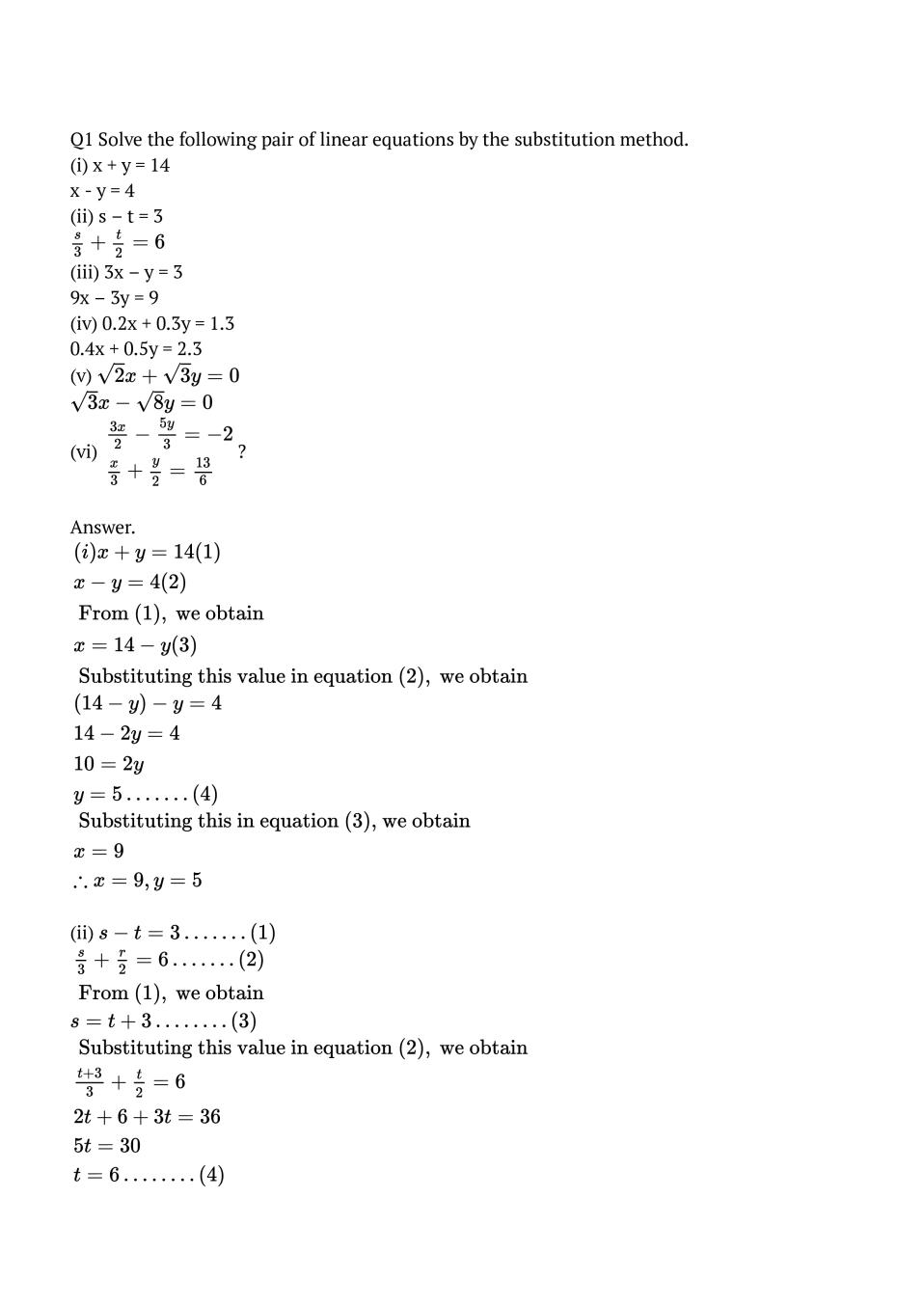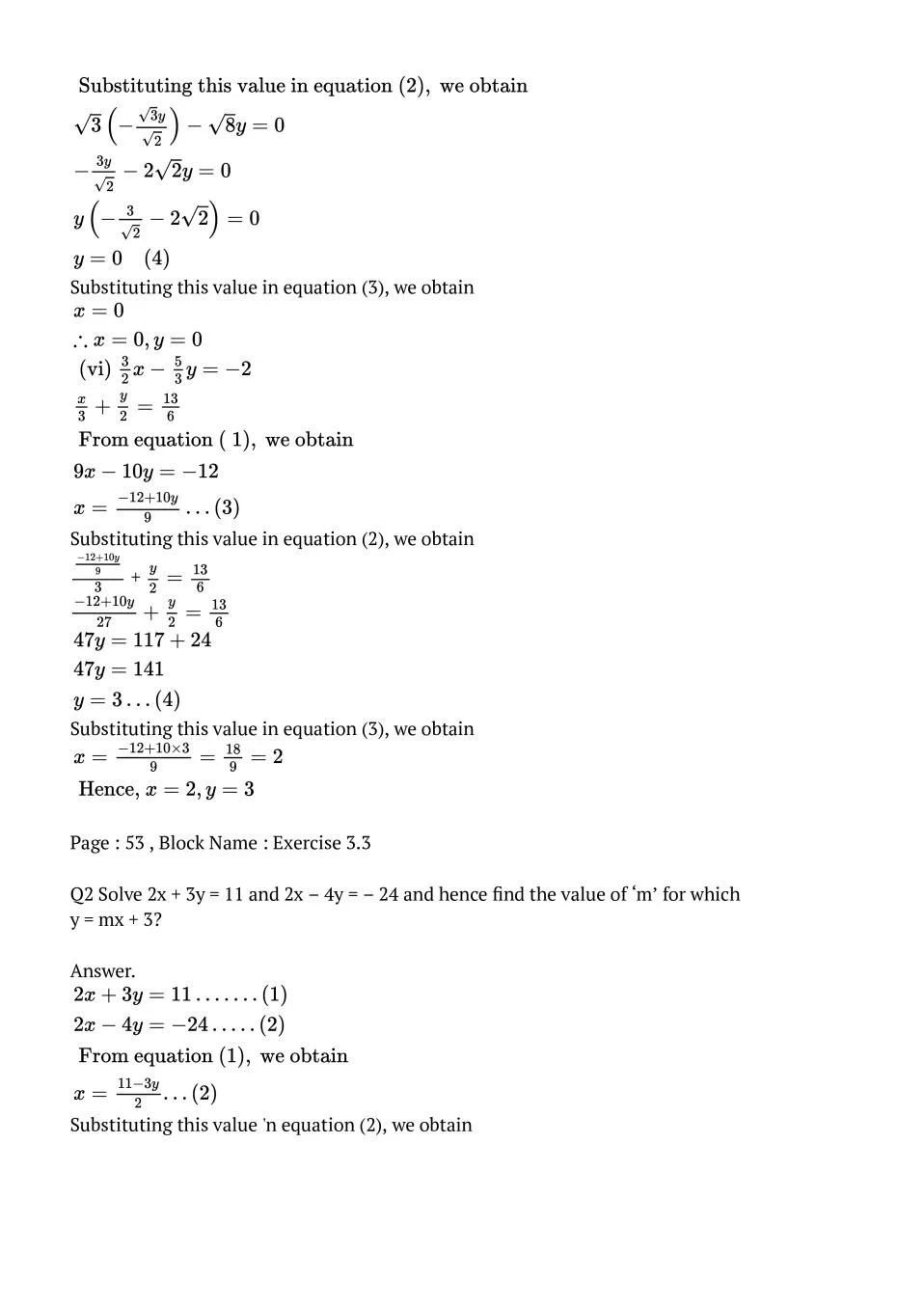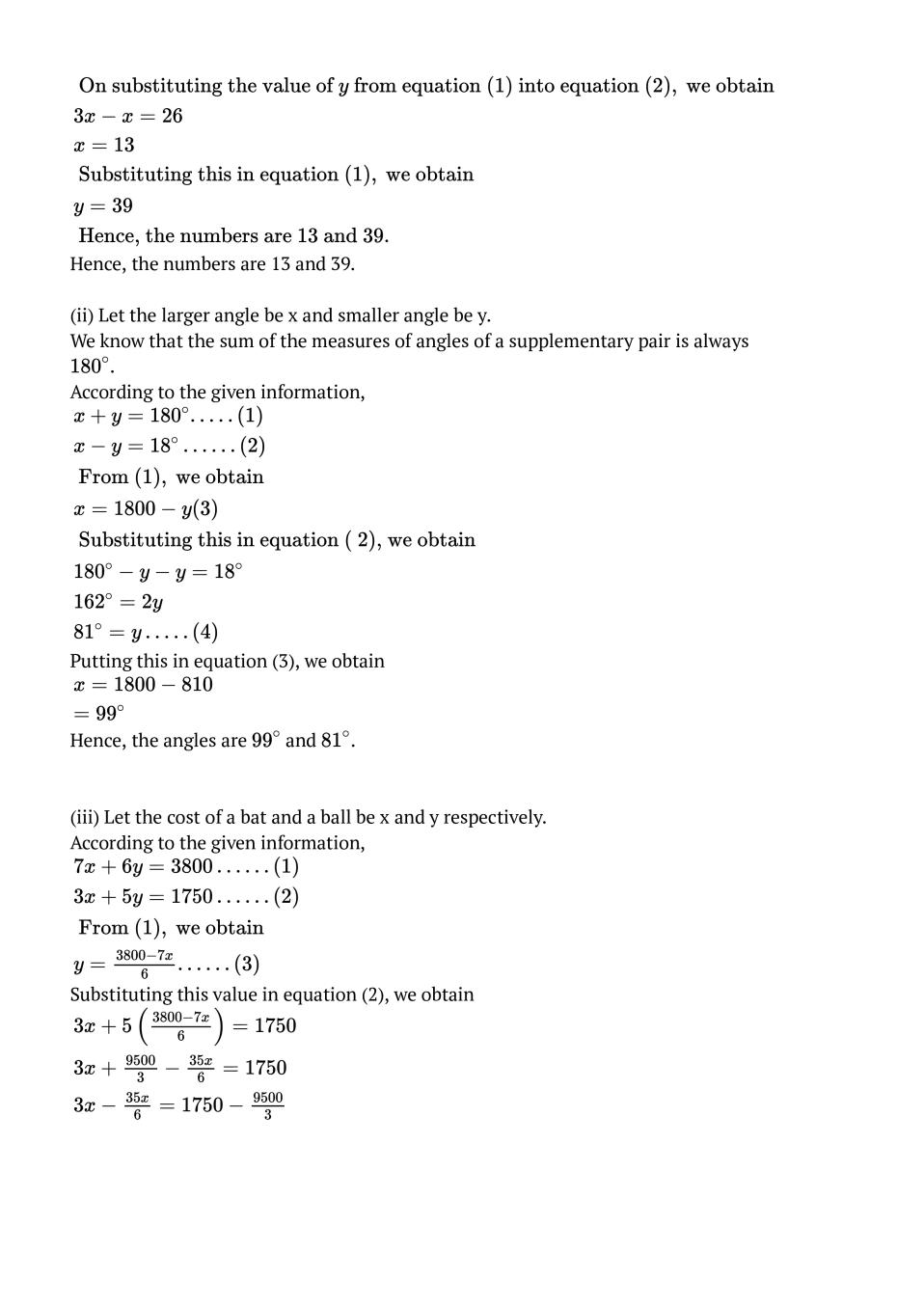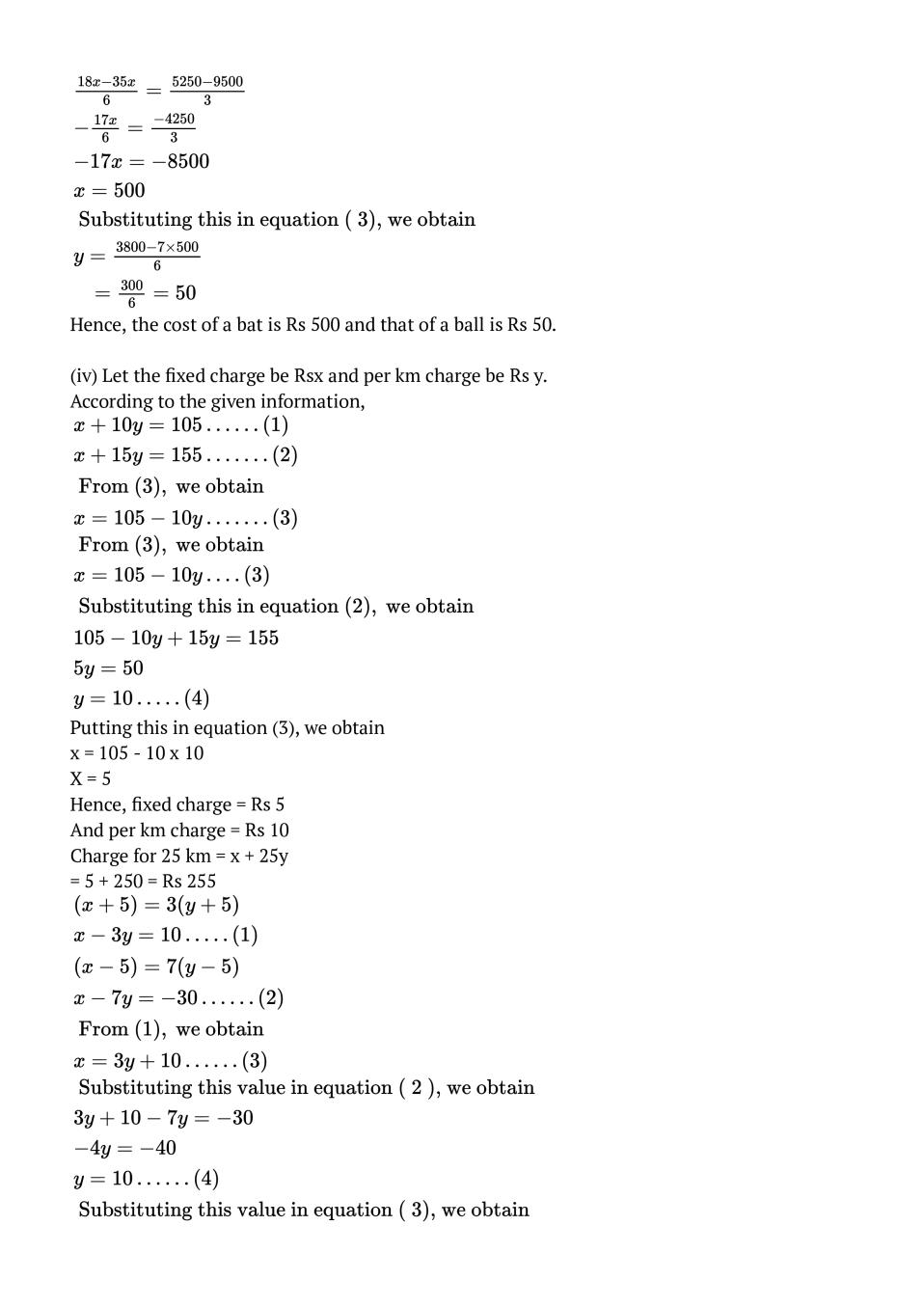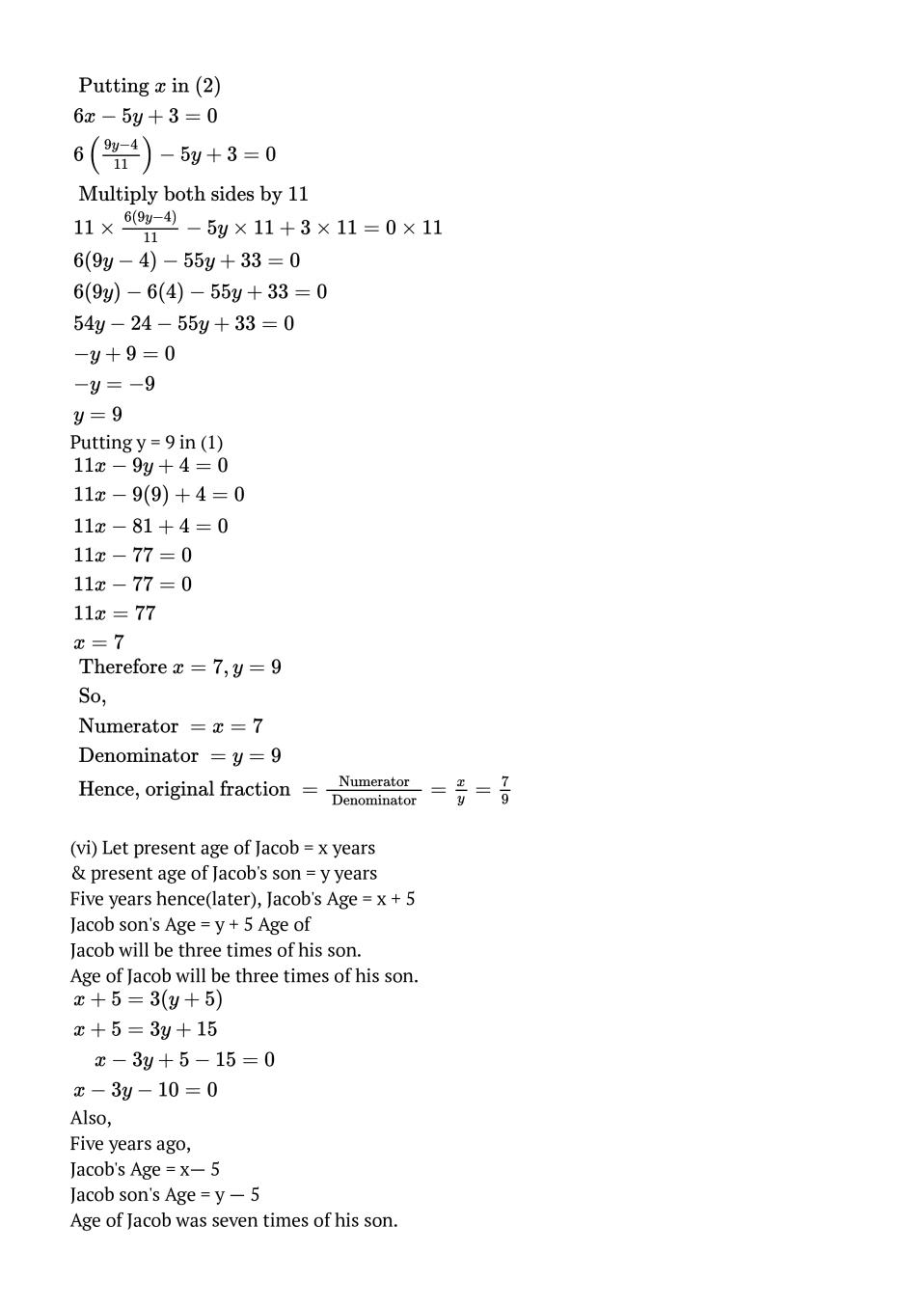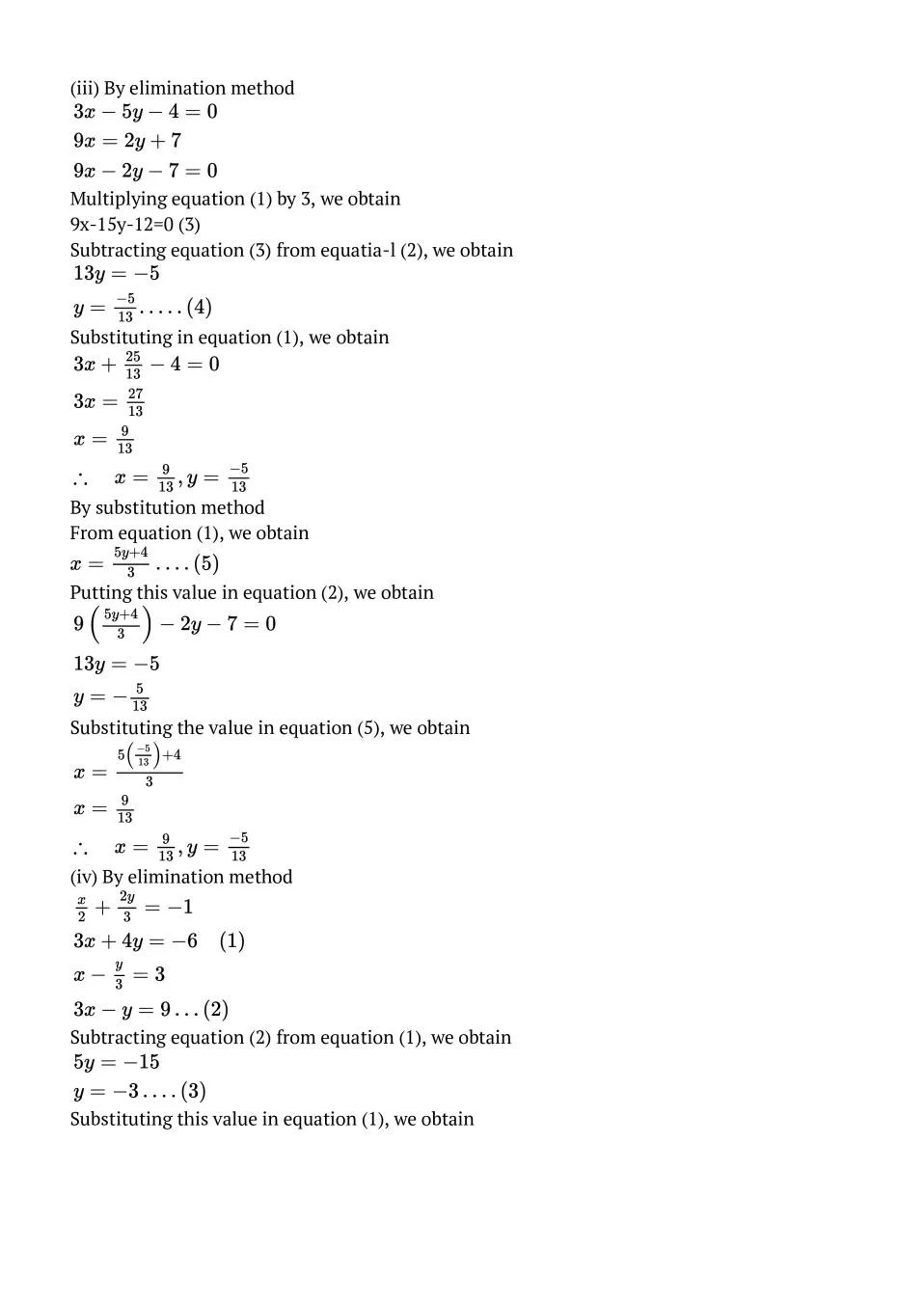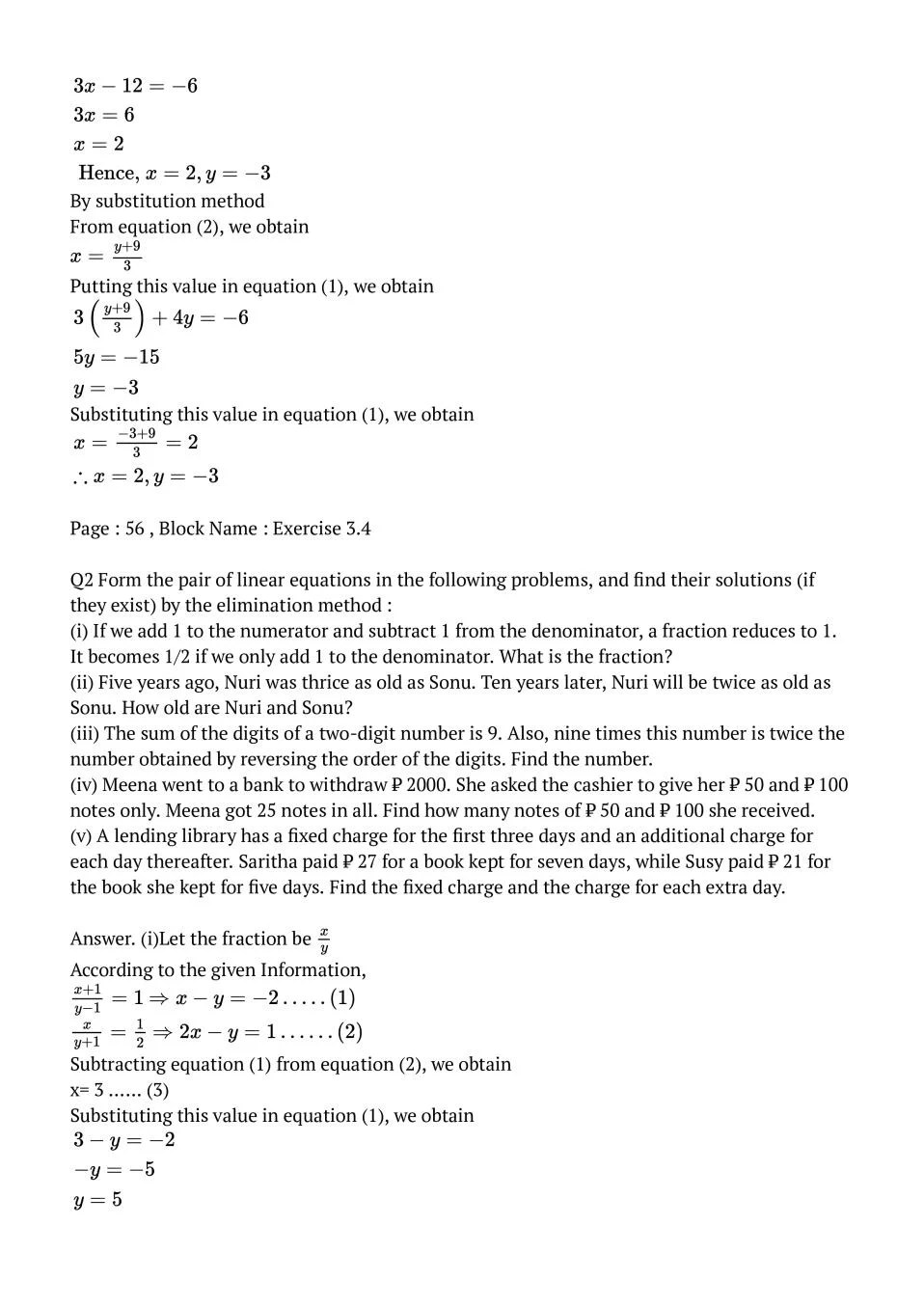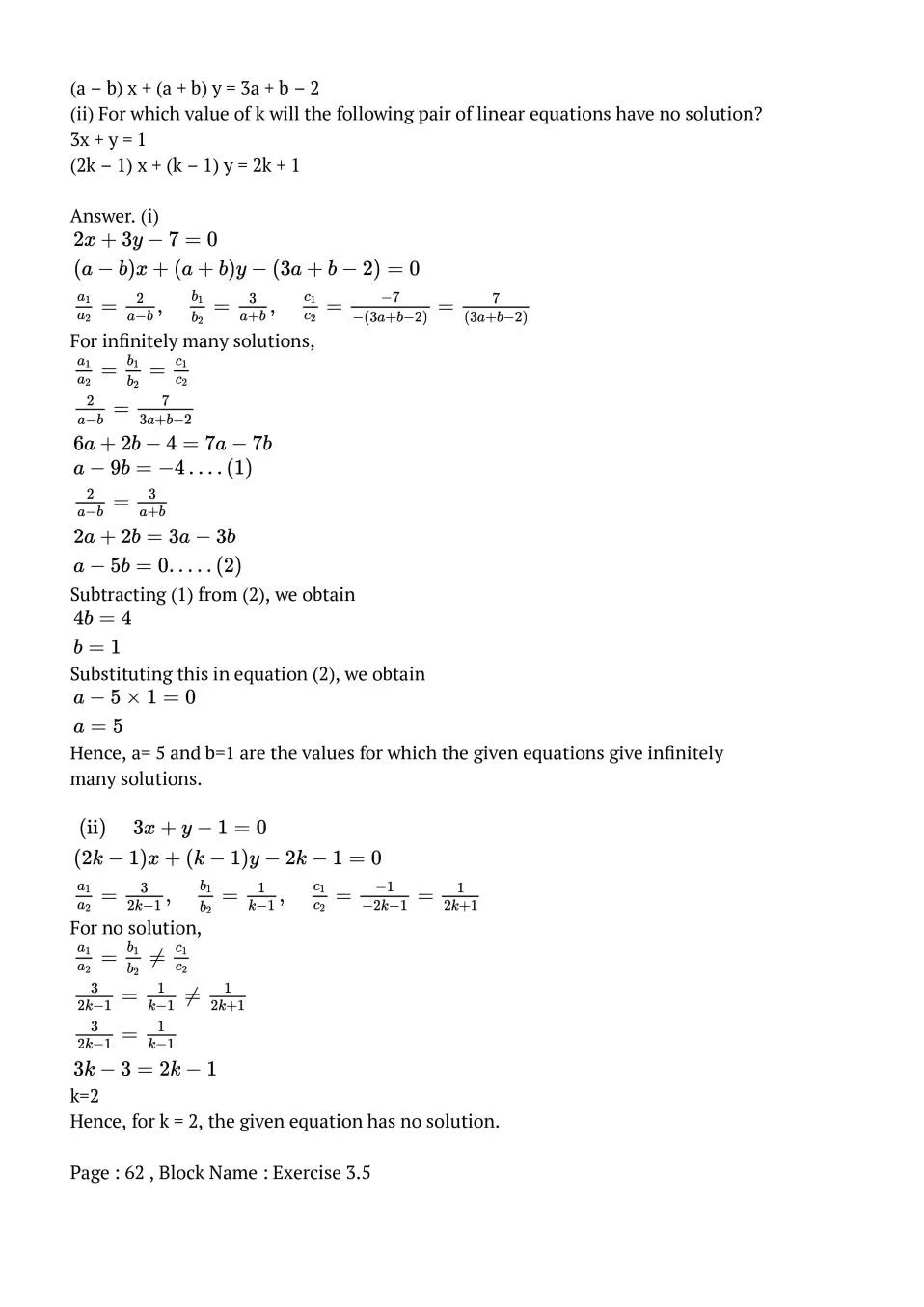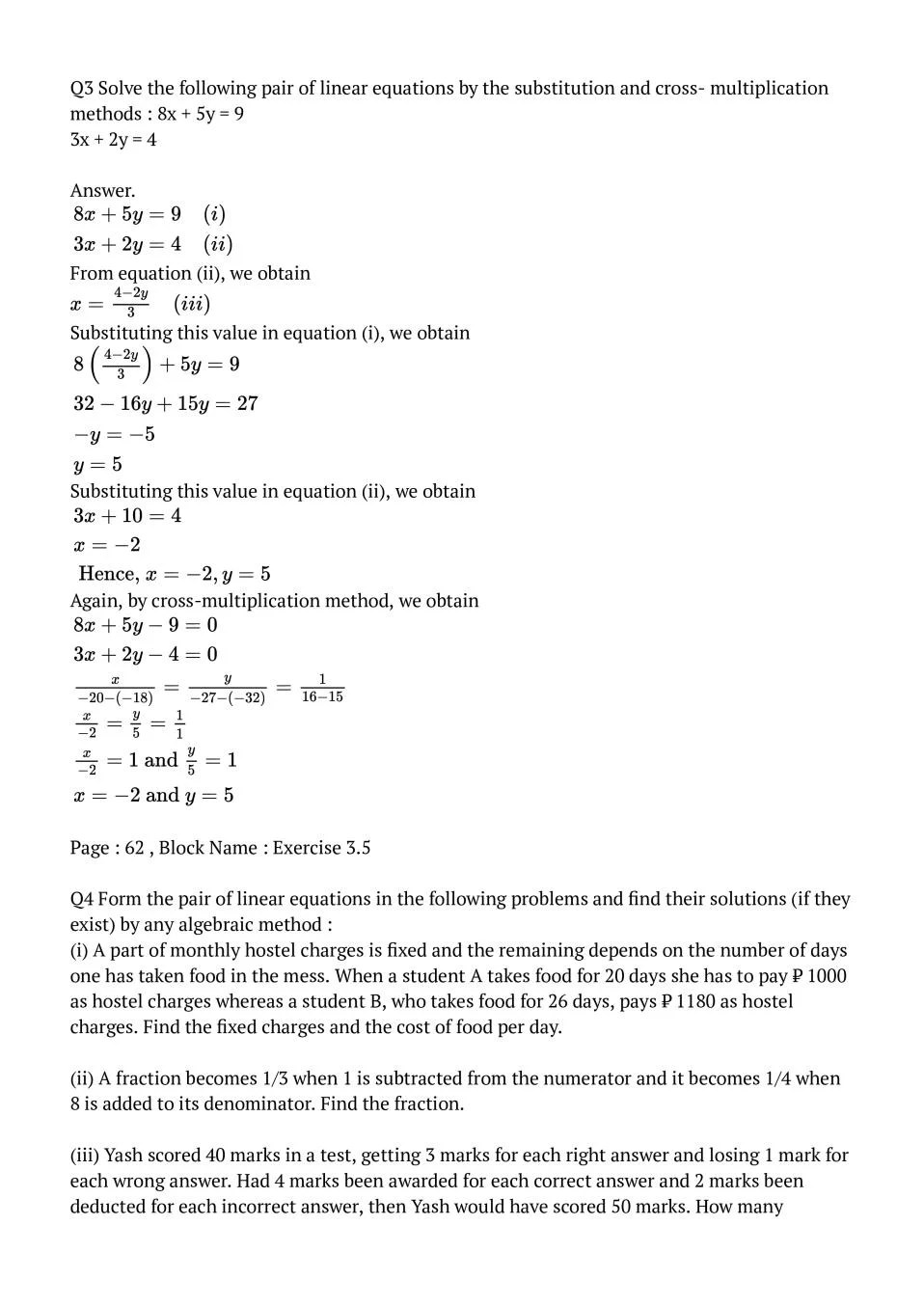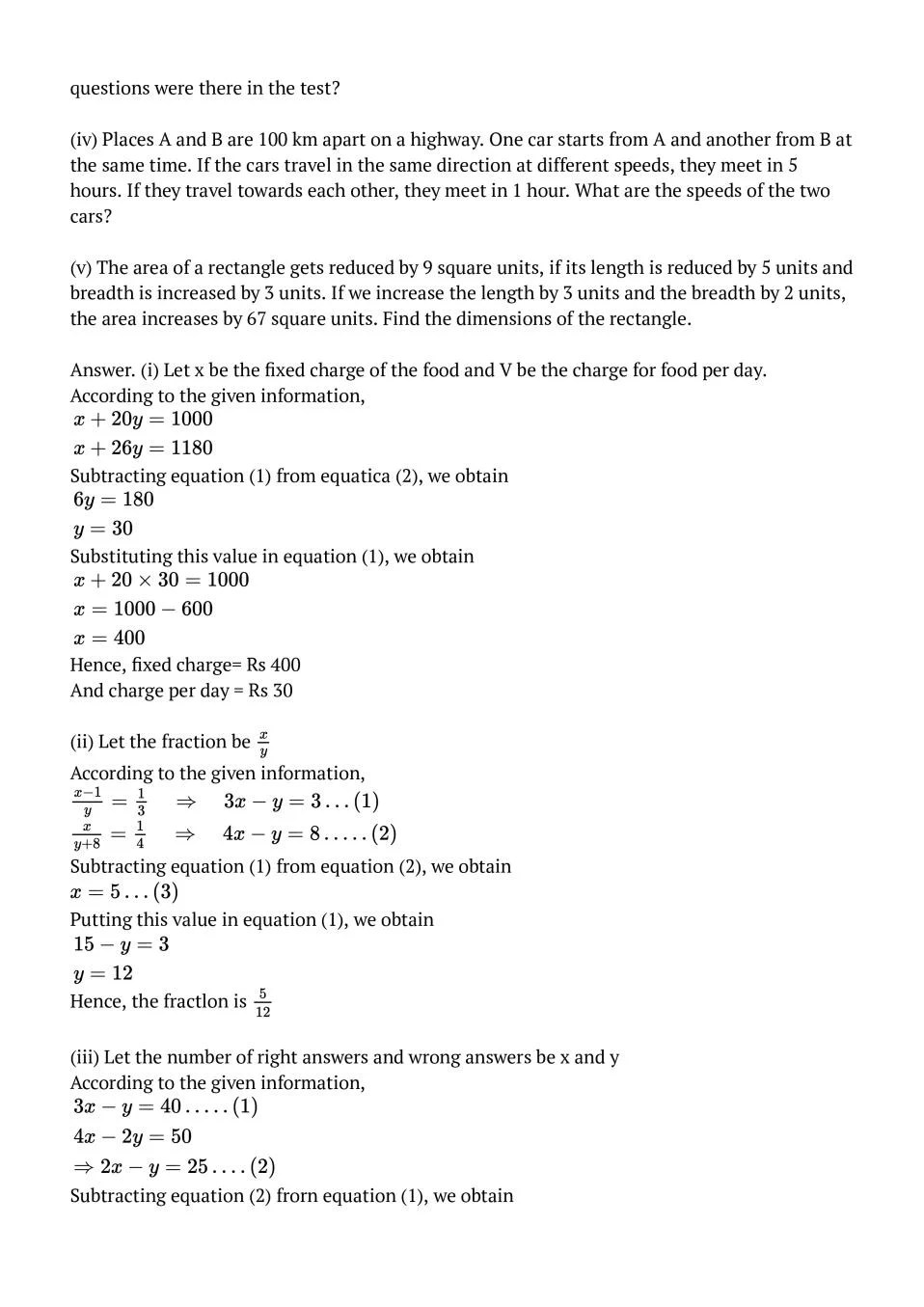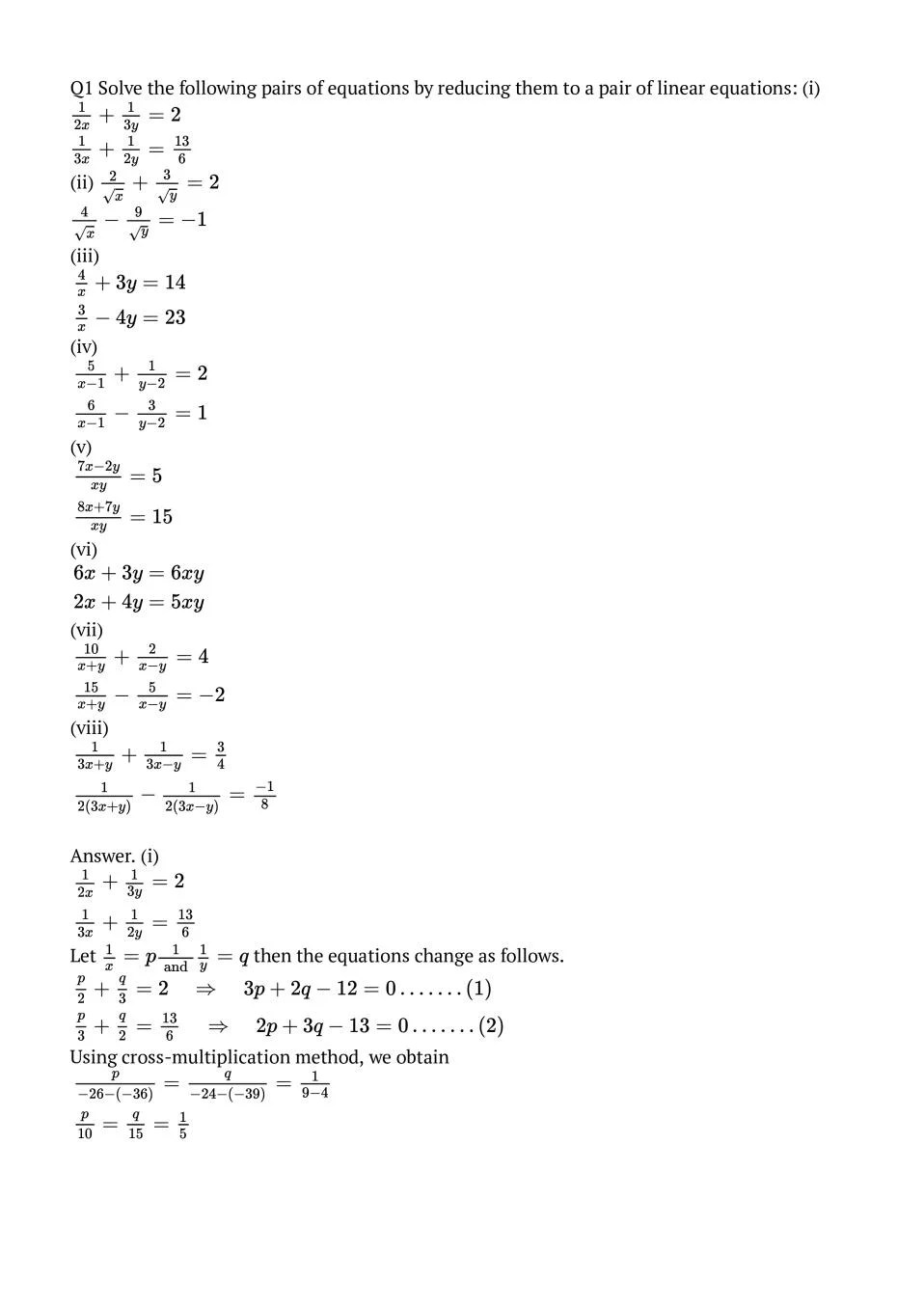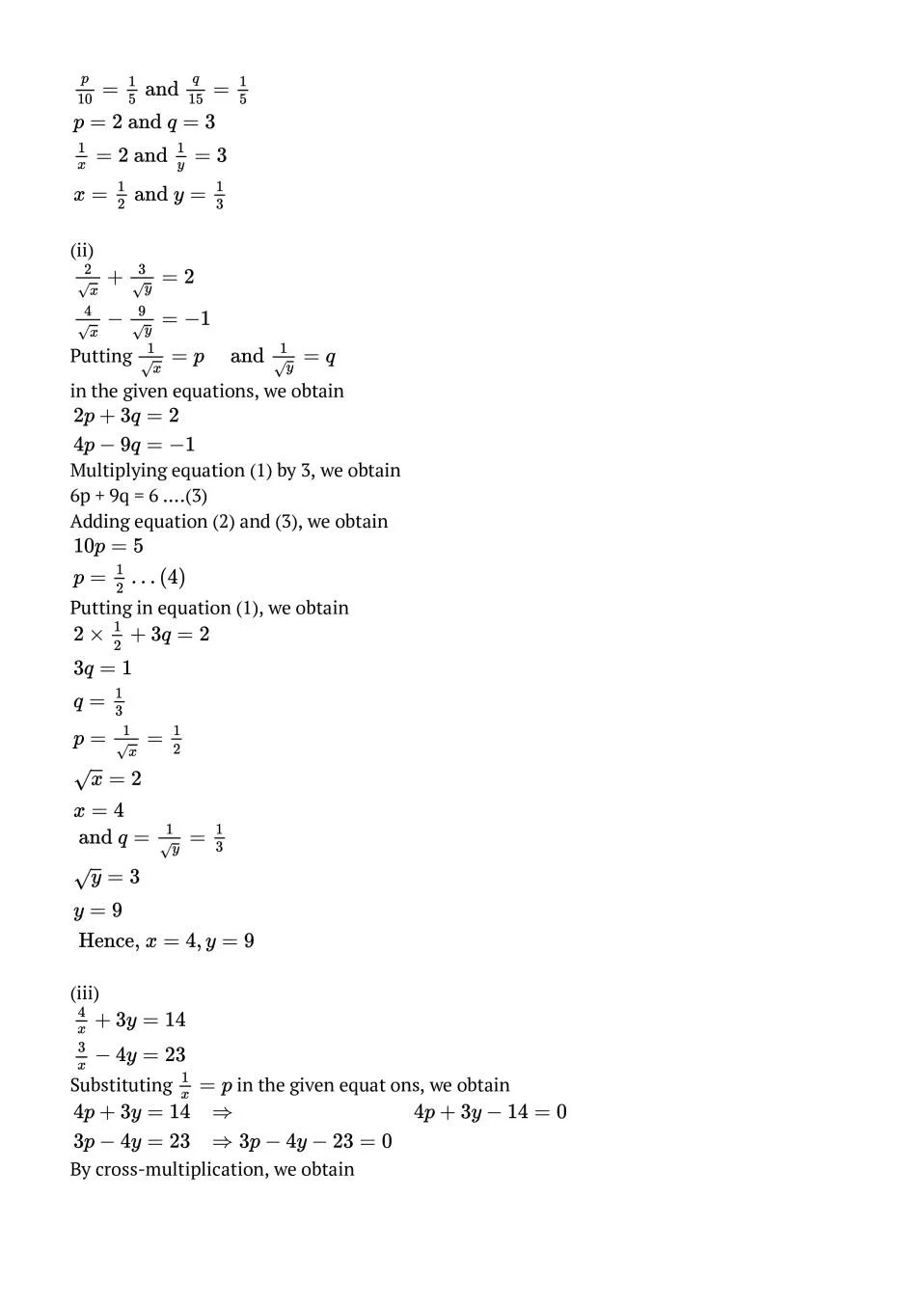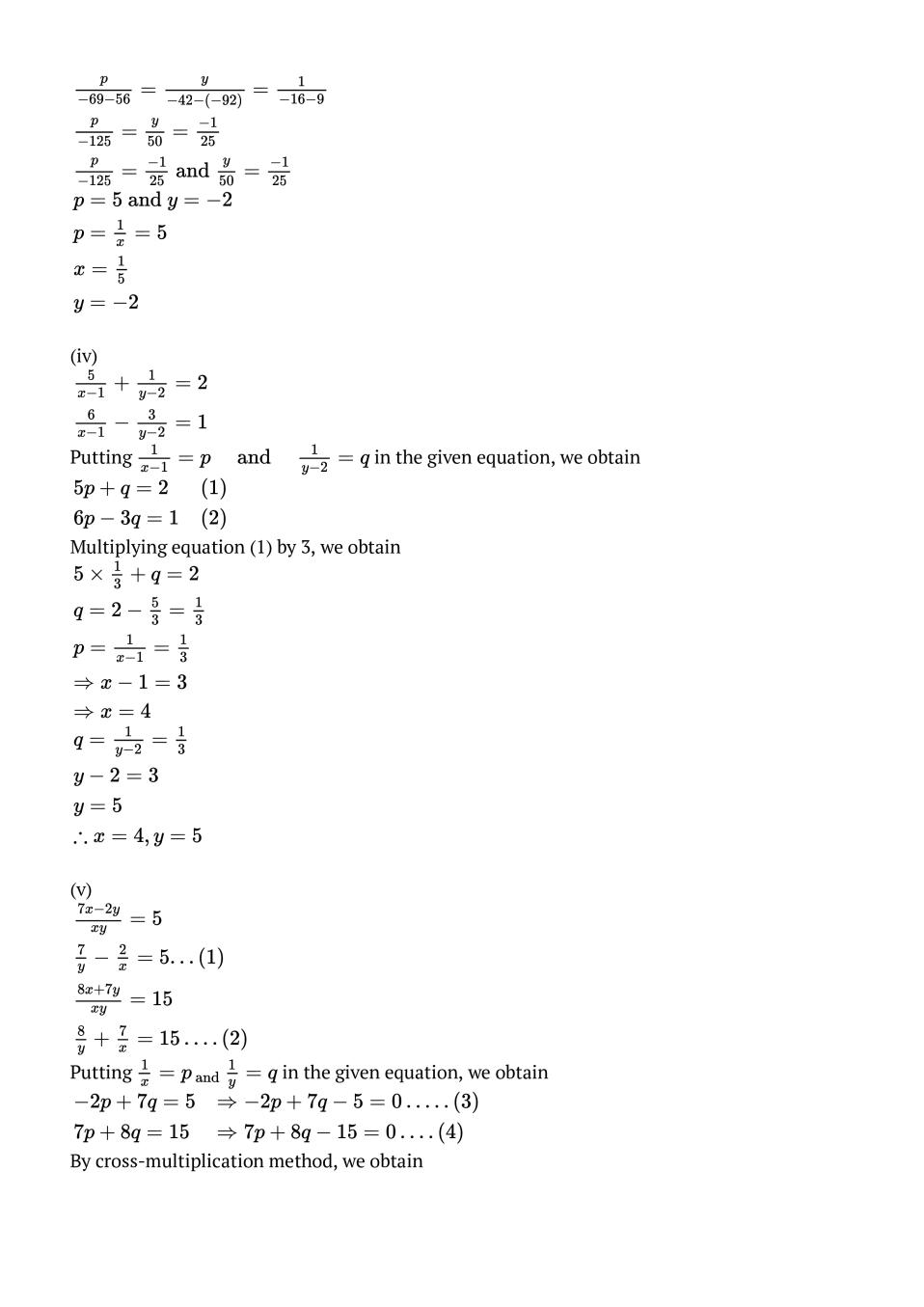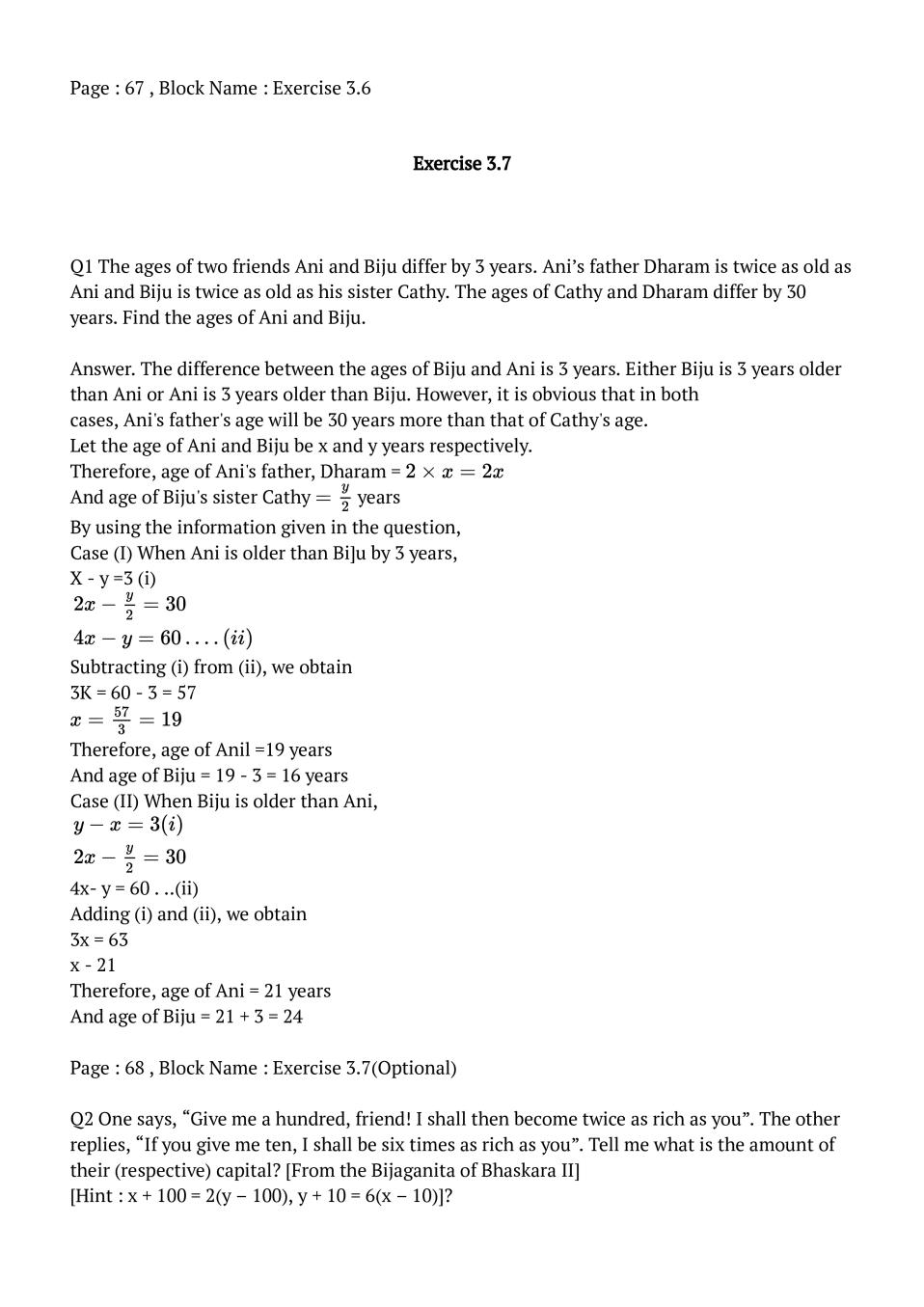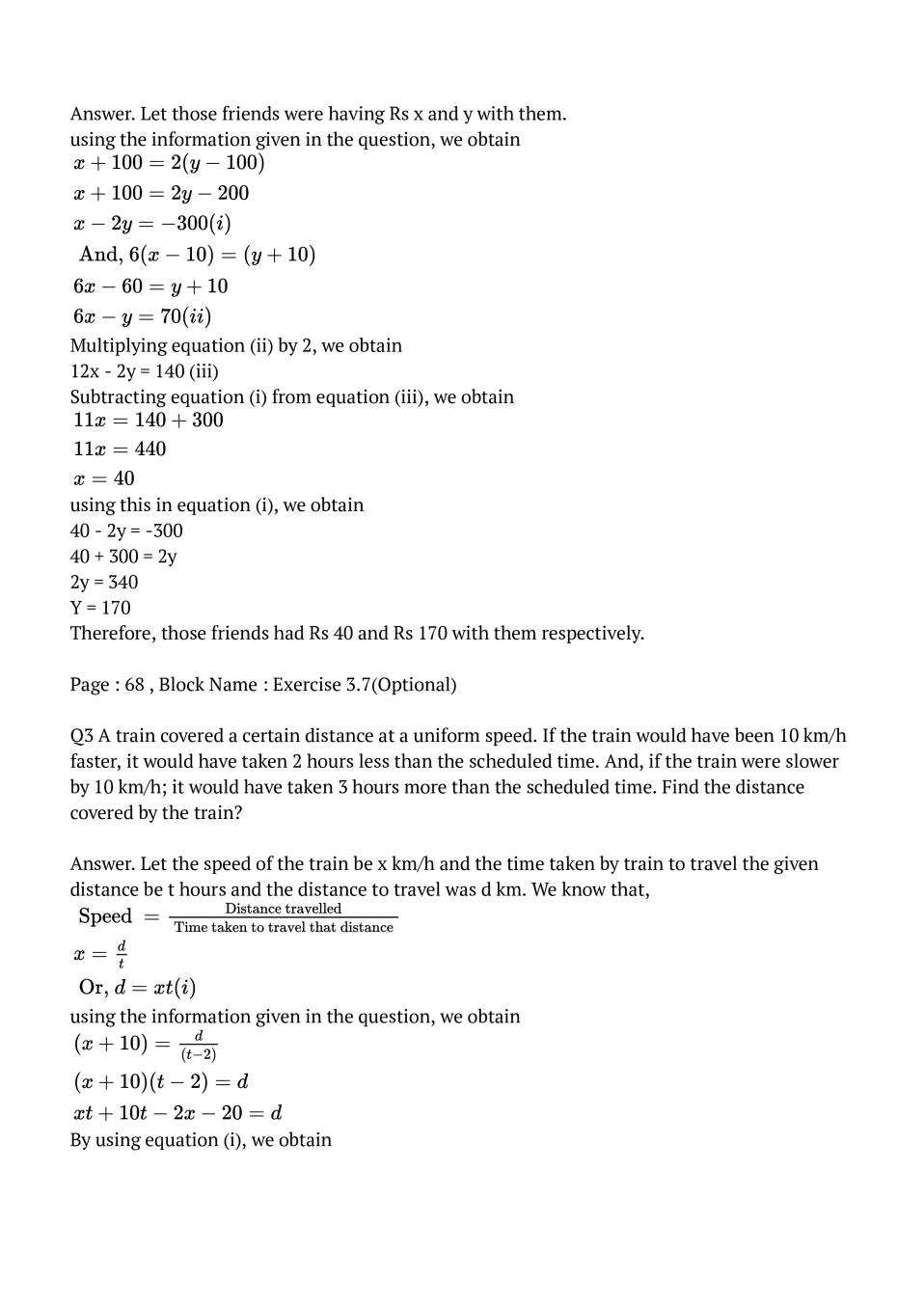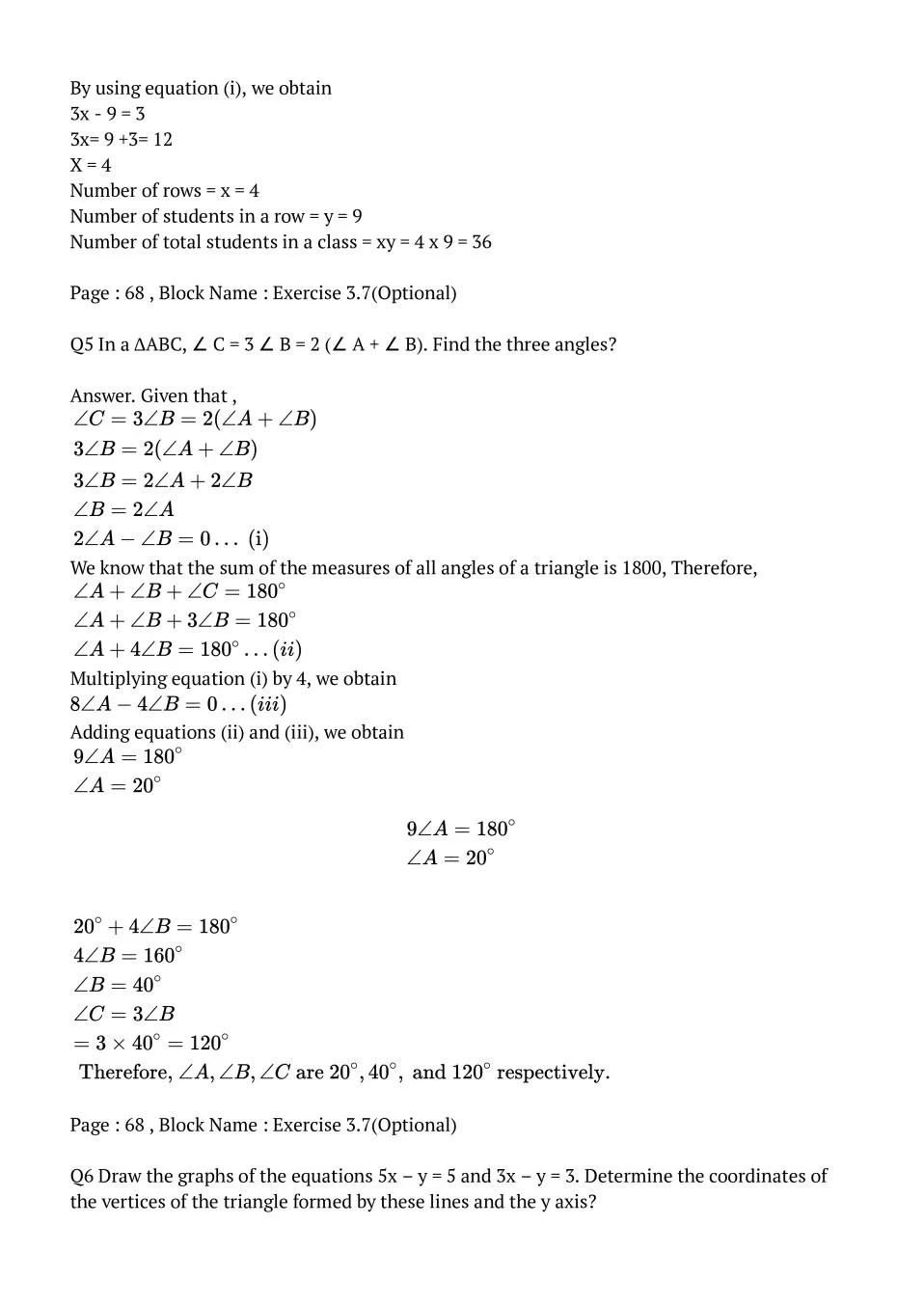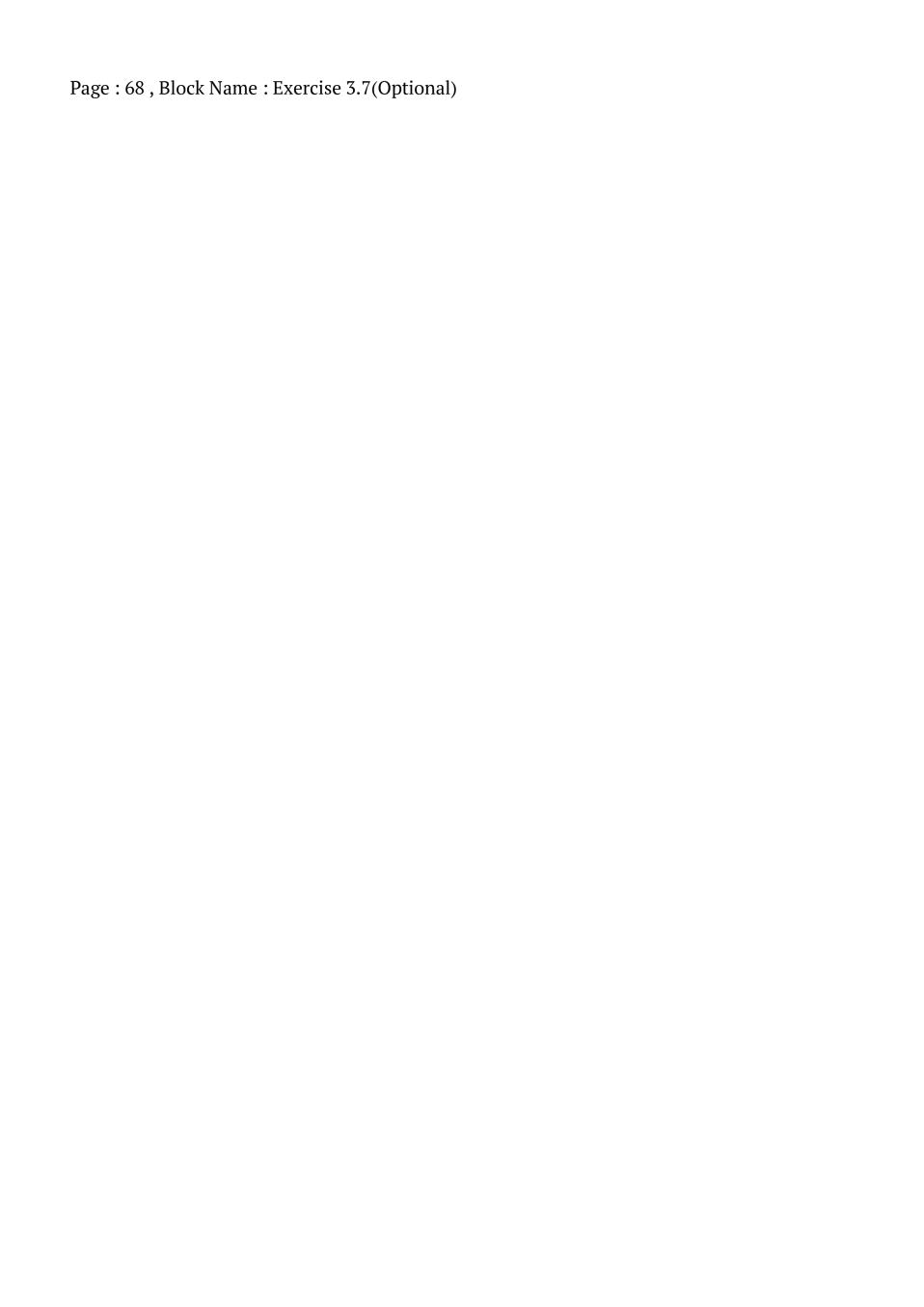### Class 10 Maths Chapter 3 NCERT Solutions

Get step by step solutions for the questions given in class 10 Maths textbook from the latest NCERT as per board exam guidelines.

### Class 10 Maths Chapter 3 Pair of Linear Equations in Two Variables Important Topics

3.1 Introduction
3.2 Pair of Linear Equations in Two Variables
3.3 Graphical Method of Solution of a Pair of Linear Equations
3.4 Algebraic Methods of Solving a Pair of Linear Equations
3.4.1 Substitution Method
3.4.2 Elimination Method
3.4.3 Cross-Multiplication Method
3.5 Equations Reducible to a Pair of Linear Equations in Two Variables
3.6 Summary

Exercise Covered under Maths Book Class 10 Maths Chapter 3 NCERT Solutions

Here on this page we have covered following exercises from NCERT Maths Book for Class 10 Maths Chapter 3 Pair Of Linear Equations In Two Variables

• Exercise: 3.1
• Exercise: 3.2
• Exercise: 3.3
• Exercise: 3.4
• Exercise: 3.5
• Exercise: 3.6
• Exercise: 3.7

We also suggest you to practice all the solved examples given in the NCERT book to clear your concepts on Pair Of Linear Equations In Two Variables. You can buy NCERT Books for Class 10 Maths online for doorstep delivery.

### NCERT Solutions Class 10 Chapter Wise

If you want NCERT solutions of any topic other than Chapter 3 Pair Of Linear Equations In Two Variables, check it from here. Maths Book by NCERT has all chapters that are in your Class 10 syllabus.

### NCERT Solutions Class 10 All Subjects

All NCERT Solutions for Subjects other than Maths are provided below:

 English Hindi Science Maths Social Science

### Other Classes NCERT Solutions

If you want NCERT solutions of any class other than Class 10, check it from here.